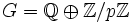# Abelian and abelian automorphism group not implies locally cyclic

## Statement

It is possible for a group$G$ to be both an abelian group and a group whose automorphism group is abelian (i.e., have an abelian automorphism group) but not be a locally cyclic group (i.e., it has a subgroup generated by two elements that is not cyclic).

## Proof

Consider the group$G = \mathbb{Q} \oplus \mathbb{Z}/2\mathbb{Z}$ where$\mathbb{Q}$ is the (additive) group of rational numbers and$\mathbb{Z}/2\mathbb{Z}$ is the cyclic group of order two and$\oplus$ denotes the external direct product.

Clearly,$G$ is abelian. We claim that the automorphism group of$G$ is abelian:

1. The first direct factor$\mathbb{Q} \oplus 0$ is characteristic in$G$: In fact, it is precisely the set of elements that are divisible by$2$, i.e., that have halves (in additive notation).
2. The second direct factor$0 \oplus \mathbb{Z}/2\mathbb{Z}$ is characteristic in$G$: In fact, it is precisely the set of elements whose order is a factor of$2$, i.e., whose double is zero (in additive notation).

Thus, an automorphism of$G$ preserves the two direct factors, and we thus get:$\operatorname{Aut}(G) \cong \operatorname{Aut}(\mathbb{Q}) \times \operatorname{Aut}(\mathbb{Z}/2\mathbb{Z})$

We know that$\operatorname{Aut}(\mathbb{Q}) \cong \mathbb{Q}^*$ is abelian, and$\operatorname{Aut}(\mathbb{Z}/2\mathbb{Z})$ is trivial, so the direct product is abelian.

Next, we claim that$G$ is not locally cyclic. To see this, note that the generator of the second direct factor has finite order and any element in the first direct factor has infinite order, so they cannot be in a common cyclic group (if the cyclic group is finite, it cannot have an element of infinite order; if it is infinite, it cannot have a nonzero element of finite order).

More generally, we can take as our$G = \mathbb{Q} \oplus \mathbb{Z}/p\mathbb{Z}$ where$p$ is a prime number.